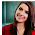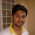# Computer System Architecture Set 30

### Questions 291 to 300

 291 Name the binary code where there is only one bit of difference between two consecutive numbers. (a) Excess-3 code                                                 (b) EBCDIC code        (c) ASCII code (d) Gray code                                                        (e) 2421 code. 292 Which one of the following is correct? (a)    Sequential circuit is an interconnection of only logic gates (b)    Sequential circuit is an interconnection of only flip flops (c)     Combinational circuit is an interconnection of logic gates (d)    Combinational circuit is an interconnection of flip flops (e)     Part of a combinational circuit is a sequential circuit. 293 SC←0, specifies_____________. (a) Seconds is assigned with 0                            (b) Signal counter is assigned with 0 (c) Sequence counter is assigned with 0           (d) Synchronous clear (e) Subroutine call. 294 __________ is the memory usually written by the manufacturer. (a) RAM                                                                 (b) ROM                        (c) DRAM           (d) SRAM                                                              (e) Cache Memory. 295 is ________________. (a) AND gate                                                         (b) OR gate                    (c) NAND gate (d) NOR gate                                                         (e) Inverted OR gate. 296 Which of the following is false related to the logic gates? (a) NOT=BUFFER                                               (b) NOR=OR+NOT (c) NAND=AND+NOT                                         (d) XNOR=XOR+NOT (e) Inverted OR=NOT+OR. 297 Transistors belong to ______ generation of the computer evolution. (a) First                           (b) Second                     (c) Third                         (d) Fourth                      (e) Fifth. 298 Identify the law for the given Boolean function, x(y+z)=xy+xz. (a) Commutative law                                          (b) Associative law      (c) Demorgan’s Law (d) Distributive law                                               (e) Involution law. 299 BCD code is also called as _________. (a) EBCDIC                  (b) 2421                         (c) Excess -3                  (d) Excess-3 gray         (e) 8421. 300 Identify the equation for the sum in the full adder. (a) A B C                                                        (b) A B                       (c) A B’ (d) A’ B’ C’                                                    (e) A’ C’.

29  30  31  32  33  34  35  36  37  38  39  Next >>

1.2.3.